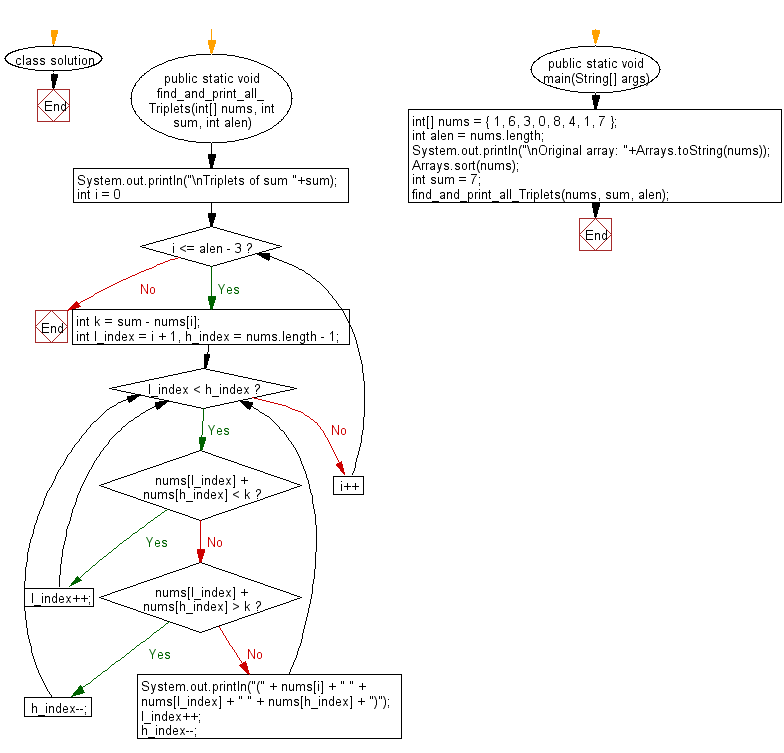﻿ Java: Find all triplets equal to a sum in a unsorted array# Java Array Exercises: Find all triplets equal to a given sum in a unsorted array of integers

## Java Array: Exercise-74 with Solution

Write a Java program to find all triplets equal to a given sum in a unsorted array of integers.

Example:
Input :
nums = { 1, 6, 3, 0, 8, 4, 1, 7 }
Output:
Triplets of sum 7
(0 1 6)
(0 3 4)

Sample Solution:

Java Code:

``````import java.util.Arrays;

class solution {

public static void find_and_print_all_Triplets(int[] nums, int sum, int alen)
{
System.out.println("\nTriplets of sum "+sum);
for (int i = 0; i <= alen - 3; i++)
{
int k = sum - nums[i];
int l_index = i + 1, h_index = nums.length - 1;

while (l_index < h_index)
{
if (nums[l_index] + nums[h_index] < k) {
l_index++;
}

else if (nums[l_index] + nums[h_index] > k) {
h_index--;
}

else {
System.out.println("(" + nums[i] + " " + nums[l_index] + " " + nums[h_index] + ")");
l_index++;
h_index--;
}
}
}
}
public static void main(String[] args)
{
int[] nums = { 1, 6, 3, 0, 8, 4, 1, 7 };
int alen = nums.length;
System.out.println("\nOriginal array: "+Arrays.toString(nums));
// sort the array in ascending order
Arrays.sort(nums);
int sum = 7;

find_and_print_all_Triplets(nums, sum, alen);
}
}
```
```

Sample Output:

```Original array: [1, 6, 3, 0, 8, 4, 1, 7]

Triplets of sum 7
(0 1 6)
(0 3 4)
```

Flowchart:Java Code Editor:

Improve this sample solution and post your code through Disqus

Previous Java Exercise: Write a Java program to sort a given array of distinct integers where all its numbers are sorted except two numbers.
Next Java Exercise: Largest gap between sorted elements of an array.

What is the difficulty level of this exercise?

Test your Programming skills with w3resource's quiz.

﻿

## Java: Tips of the Day

countOccurrences

Counts the occurrences of a value in an array.

Use Arrays.stream().filter().count() to count total number of values that equals the specified value.

```public static long countOccurrences(int[] numbers, int value) {
return Arrays.stream(numbers)
.filter(number -> number == value)
.count();
}
```

Ref: https://bit.ly/3kCAgLb